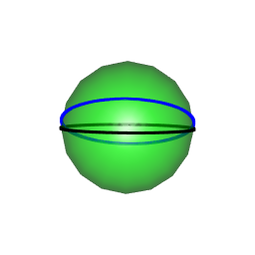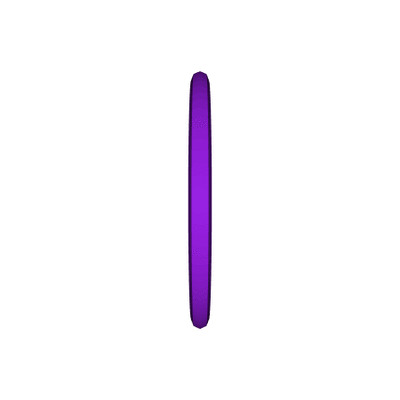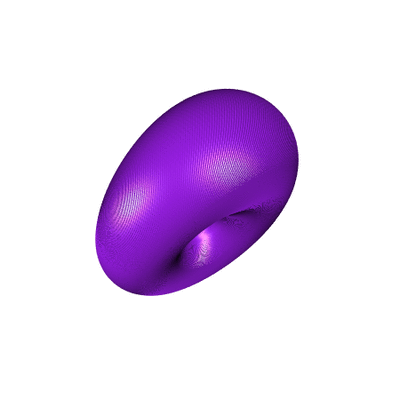# Hopf Torus (2/3): the bent equatorial case

Posted on May 1, 2018 by Stéphane Laurent
Tags: R, graphics, rgl

In this second part, we will see what happens when we map the points lying on a bent equator.

hopfinverse <- function(q, t){
1/sqrt(2*(1+q)) * c(q*cos(t)+q*sin(t),
sin(t)*(1+q),
cos(t)*(1+q),
q*sin(t)-q*cos(t))
}
stereog <- function(x){
c(x, x, x)/(1-x)
}

The sphere with the bent equator will be plotted thanks to the following functions.To rotate the equator to the bent equator, we used the rotation matrix $R_x = \begin{pmatrix} 1 & 0 & 0 \\ 0 & \cos \alpha & -\sin \alpha \\ 0 & \sin \alpha & \cos \alpha \end{pmatrix}$

Rx <- function(alpha) {
rbind(c(1, 0, 0),
c(0, cos(alpha), -sin(alpha)),
c(0, sin(alpha), cos(alpha)))
}

Now, let’s see the Hopf torus.

open3d(windowRect=c(50,50,500,500))
view3d(45,45)
t_ <- seq(0, 2*pi, len=200)
theta_ <- seq(0, 2*pi, len=300)
phi <- 0
for(i in seq_along(theta_)){
theta <- theta_[i]
rotated <- c(Rx(-pi/8) %*% c(cos(theta)*cos(phi), sin(theta)*cos(phi), sin(phi)))
circle4d <- sapply(t_, function(t){
hopfinverse(rotated, t)
})
circle3d <- t(apply(circle4d, 2, stereog))
}